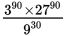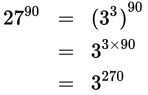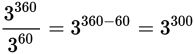# SAT Math Multiple Choice Question 569: Answer and Explanation

### Test Information

Question: 569

14. What is the value of?

• A. 95
• B. 915
• C. 930
• D. 9150

Explanation:

D

Difficulty: Hard

Category: Passport to Advanced Math / Exponents

Strategic Advice: The numbers in some questions are simply too large to use a calculator (you get an "overflow" error message). Instead, you'll have to rely on rules of exponents.

Getting to the Answer: Notice that all of the base numbers have 3 as a factor, so rewrite everything in terms of 3. This will allow you to use the rules of exponents. Because 27 is the cube of 3, you can rewrite 2790 as a power of 3. Then you can use rules of exponents to simplify:Now the numerator should read: 390 × 3270, which is equal to 390+270 = 3360. Repeat this process for the denominator:

930 = (32)30 = 32×30 = 360

Finally, use rules of exponents one more time to simplify the new expression:All the answer choices are given as powers of 9, so rewrite your answer as a power of 9:

3300 = 32×150 = (32)150 = 9150